6.12 Circles - Part 3:

6.12.1: Arcs of a circle

Two arcs of two different  circles having same radii are said to be ‘congruent’ if their central angles are same.

Arc ASB = Arc CTD if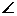AOB =CO’D

6.12.1 Theorem 1: If two arcs are congruent then their chords are equal

To prove: AB=CD

Proof:

 1. OA = O’C, OB = O’D (Radii) 2.AOB =CO’D (it is given that arcs are congruent) Hence by SAS Postulate on congruence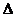AOB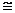CO’D Hence AB = CD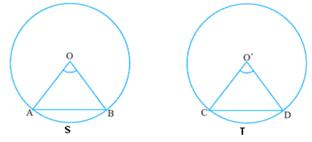6.12.1 Theorem 2: If two chords of circles having same radii are same,

then their arcs are congruent.

Note: This is converse of the previous theorem.

Use SSS postulate to show thatAOB =CO’D

6.12.1: Areas of sectors/segments of circle

 If ‘r’ is the radius of a circle, we know that the circumference and area of the circle are given by Circumference of the circle = 2r, Area of the circle =r2,                                  Whereis a constant whose approximate value we use for our calculations is 22/7 (3.1428). If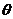(whereis in degrees) is the angle at center (COD) formed by the arc CSD then 1. Length of the arc CSD = (/180) *r 2. Area of the sector CSDO (shaded portion in the adjoining figure) = (/360) *r2 = (/180) *(r*r)/2 = {(/180) *r}*(r/2) = Length of the arc*(radius/2) Note:radians = 1800 and x0 = (x*)/180 radians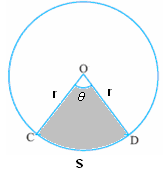LetAOB =in the adjoining figure with AB as chord We note that Area of triangle ABO = (1/2)*base*height = (1/2)*BO*AM = (1/2) *r*rsin= (1/2) r2*sin(AM = rsin: Refer to section 7.1 for definition of sin of an angle)   From the figure we notice that Area of Sector ASBO = Area of triangle ABO + Area of segment ASBArea of segment ASB = Area of Sector ASBO - Area of triangle ABO = (/360) *r2 - (1/2) r2*sin= r2 {(*/360) - (sin/2)} Note: For all the above calculationsmust be in degrees.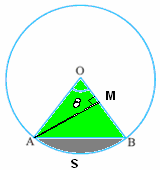6.12 Summary of learning

 No Points to remember 1 Congruency of arcs 2 Formula for length of an arc, area of an arc, Area of a segment